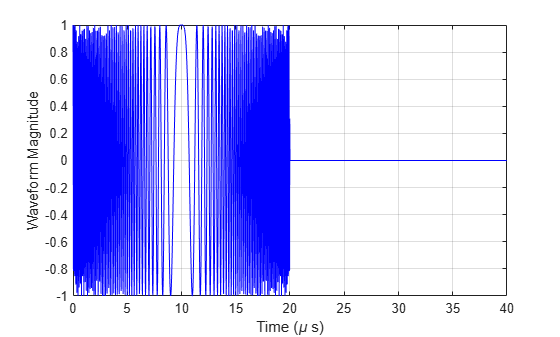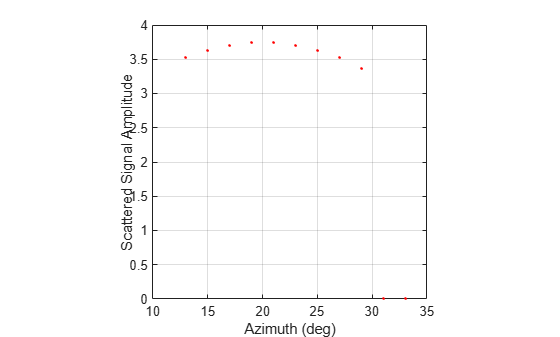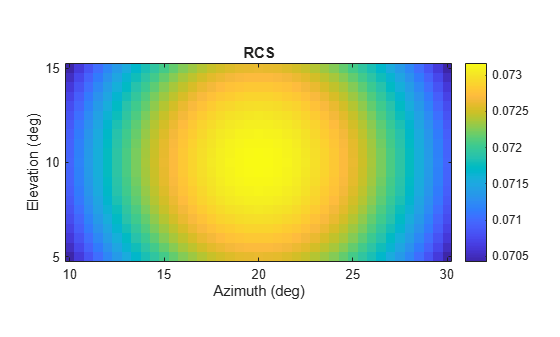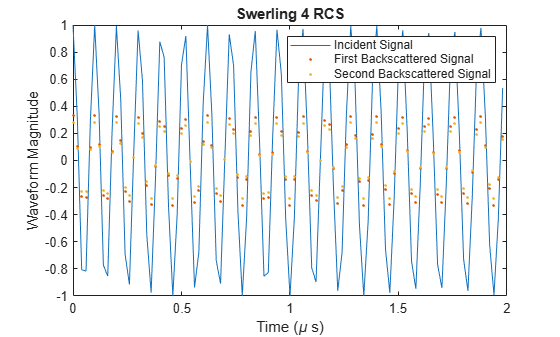Backscatter wideband signal from radar target

## Description

The phased.WidebandBackscatterRadarTarget System object™ models the backscattering of a wideband signal from a target. Backscattering is a special case of radar target scattering where the incident and reflected angles are the same. This type of scattering applies to monostatic radar configurations. The radar cross-section determines the backscattering response of a target to an incoming signal. This System object lets you specify an angle-dependent radar cross-section model that covers a range of incident angles. The wideband signal is decomposed into frequency subbands which are backscattered independently and then recombined.

This System object creates a backscattered signal for polarized or nonpolarized signals. Although electromagnetic radar signals are polarized, you can often ignore polarization in your simulation and process the signals as scalars. To ignore polarization, specify the EnablePolarization property as false. To employ polarization, specify EnablePolarization as true.

For nonpolarized signals, specify the radar cross section (RCS) as an array of values at discrete azimuth and elevation angles and discrete frequencies. The System object interpolates values for incident angles between array points. For polarized signals, specify the radar scattering matrix (SCM) using three arrays defined at discrete azimuth and elevation angles and discrete frequencies. These three arrays correspond to the HH, HV, and VV polarization components. The VH component is computed from the conjugate symmetry of the HV component. H and V stand for the horizontal and vertical polarization components, respectively.

For both nonpolarized and polarized signals, you can employ one of four Swerling models to generate random fluctuations in the RCS or radar scattering matrix. Choose which model using the Model property. Then, use the SeedSource and Seed properties to randomize the fluctuations.

EnablePolarizationRadar cross-section patterns
false

RCSPattern

trueShhPattern, SvvPattern, and ShvPattern

To compute the propagation delay for specified source and receiver points:

1. Define and set up your radar target. You can set phased.WidebandBackscatterRadarTarget System object properties at construction time or leave them set to their default values. See Construction. Some properties that you set at construction time can be changed later. These properties are tunable.

2. To compute the propagated signal, call the step method . The output of the method depends on the properties of the object. You can change tunable properties at any time.

Note

Alternatively, instead of using the step method to perform the operation defined by the System object, you can call the object with arguments, as if it were a function. For example, y = step(obj,x) and y = obj(x) perform equivalent operations.

## Construction

target = phased.WidebandBackscatterRadarTarget creates a wideband backscatter radar target System object, target.

target = phased.WidebandBackscatterRadarTarget(Name,Value) creates a wideband backscatter radar target object, with each specified property Name set to the specified Value. You can specify additional name and value pair arguments in any order as (Name1,Value1,...,NameN,ValueN).

## Properties

expand all

Option to enable processing of polarized signals, specified as false or true. Set this property to true to allow the target to simulate the reflection of polarized radiation. Set this property to false to ignore polarization.

Example: true

Specify the wideband backscatter pattern frequencies used in the RCS or SCM matrices. The elements of this vector must be in strictly increasing order. The target has no response outside this frequency range. Frequencies are defined with respect to the physical frequency band, not the baseband. Frequency units are in hertz.

Azimuth angles used to define the angular coordinates of each column of the matrices specified by the RCSPattern, ShhPattern, ShvPattern, or SvvPattern properties. Specify the azimuth angles as a length P vector. P must be greater than two. Angle units are in degrees.

Example: [-45:0.1:45]

Data Types: double

Elevation angles used to define the angular coordinates of each row of the matrices specified by the RCSPattern, ShhPattern, ShvPattern, or SvvPattern properties. Specify the elevation angles as a length Q vector. Q must be greater than two. Angle units are in degrees.

Example: [-30:0.1:30]

Data Types: double

Radar cross-section pattern, specified as a real-valued matrix or array.

DimensionsApplication
Q-by-P matrixSpecifies a matrix of RCS values as a function of Q elevation angles and P azimuth angles. The same RCS matrix is used for all frequencies.
Q-by-P-by-K arraySpecifies an array of RCS patterns as a function of Q elevation angles, P azimuth angles, and K frequencies. If K = 1, the RCS pattern is equivalent to a Q-by-P matrix.
1-by-P-by-K arraySpecifies a matrix of RCS values as a function of P azimuth angles and K frequencies. These dimension formats apply when there is only one elevation angle.
K-by-P matrix

• Q is the length of the vector specified by the ElevationAngles property.

• P is the length of the vector specified by the AzimuthAngles property.

• K is the number of frequencies specified by the FrequencyVector property.

You can specify patterns for L targets by putting L patterns into a cell array. All patterns must have the same dimensions. The value of L must match the column dimensions of the signals passed as input into the step method. However, you can use one pattern to model L multiple targets.

RCS units are in square meters.

Example: [1,1;1,1]

#### Dependencies

To enable this property, set the EnablePolarization property to false.

Data Types: double

Radar scattering matrix (SCM) HH polarization component, specified as a complex-valued matrix or array.

DimensionsApplication
Q-by-P matrixSpecifies the scattering matrix polarization component as a function of Q elevation angles and P azimuth angles. The same SCM matrix is used for all frequencies.
Q-by-P-by-K arraySpecifies the scattering matrix polarization component as a function of Q elevation angles, P azimuth angles, and K frequencies. If K = 1, the RCS pattern is equivalent to a Q-by-P matrix.
1-by-P-by-K arraySpecifies the scattering matrix polarization component as a function of P azimuth angles and K frequencies. These dimension formats apply when there is only one elevation angle.
K-by-P matrix

• Q is the length of the vector specified by the ElevationAngles property.

• P is the length of the vector specified by the AzimuthAngles property.

• K is the number of frequencies specified by the FrequencyVector property.

You can specify polarization component patterns for L targets by putting L patterns into a cell array. All patterns must have the same dimensions. The value of L must match the column dimensions of the signals passed as input into the step method. You can, however, use one pattern to model L multiple targets.

SCM units are in square-meters.

Example: [1,1;1i,1i]

#### Dependencies

To enable this property, set the EnablePolarization property to true.

Data Types: double
Complex Number Support: Yes

Radar scattering matrix VV-pol component, specified as a complex-valued vector, matrix, or array. Different dimension cases have different applications.

DimensionsApplication
Q-by-P matrixSpecifies the scattering matrix polarization component as a function of Q elevation angles and P azimuth angles. The same SCM matrix is used for all frequencies.
Q-by-P-by-K arraySpecifies the scattering matrix polarization component as a function of Q elevation angles, P azimuth angles, and K frequencies. If K = 1, the RCS pattern is equivalent to a Q-by-P matrix.
1-by-P-by-K arraySpecifies the scattering matrix polarization component as a function of P azimuth angles and K frequencies. These dimension formats apply when there is only one elevation angle.
K-by-P matrix

• Q is the length of the vector specified by the ElevationAngles property.

• P is the length of the vector specified by the AzimuthAngles property.

• K is the number of frequencies specified by the FrequencyVector property.

You can specify polarization component patterns for L targets by putting L patterns into a cell array. All patterns must have the same dimensions. The value of L must match the column dimensions of the signals passed as input into the step method. You can, however, use one pattern to model L multiple targets.

SCM units are in square-meters.

Example: [1,1;1i,1i]

#### Dependencies

To enable this property, set the EnablePolarization property to true.

Data Types: double
Complex Number Support: Yes

Radar scattering matrix (SCM) HV-pol component, specified as a complex-valued vector, matrix, or array. Different dimension cases have different applications.

DimensionsApplication
Q-by-P matrixSpecifies the scattering matrix polarization component as a function of Q elevation angles and P azimuth angles. The same SCM matrix is used for all frequencies.
Q-by-P-by-K arraySpecifies the scattering matrix polarization component as a function of Q elevation angles, P azimuth angles, and K frequencies. If K = 1, the RCS pattern is equivalent to a Q-by-P matrix.
1-by-P-by-K arraySpecifies the scattering matrix polarization component as a function of P azimuth angles and K frequencies. These dimension formats apply when there is only one elevation angle.
K-by-P matrix

• Q is the length of the vector specified by the ElevationAngles property.

• P is the length of the vector specified by the AzimuthAngles property.

• K is the number of frequencies specified by the FrequencyVector property.

You can specify polarization component patterns for L targets by putting L patterns into a cell array. All patterns must have the same dimensions. The value of L must match the column dimensions of the signals passed as input into the step method. You can, however, use one pattern to model L multiple targets.

SCM units are in square-meters.

Example: [1,1;1i,1i]

#### Dependencies

To enable this property, set the EnablePolarization property to true.

Data Types: double
Complex Number Support: Yes

Target fluctuation model, specified as 'Nonfluctuating', 'Swerling1', 'Swerling2', 'Swerling3', or 'Swerling4'. If you set this property to a value other than 'Nonfluctuating', use the update input argument when calling the step method.

Example: 'Swerling3'

Data Types: char

Signal propagation speed, specified as a positive scalar. Units are in meters per second. The default propagation speed is the value returned by physconst('LightSpeed'). See physconst for more information.

Example: 3e8

Data Types: double

Operating frequency, specified as a positive scalar. Units are in Hz.

Example: 1e9

Data Types: double

Signal sample rate, specified as a positive real-valued scalar. Units are in hertz.

Example: 1e6

Data Types: double

Number of processing subbands, specified as a positive integer.

Example: 128

Data Types: double

Seed source of random number generator for RCS fluctuation model, specified as 'Auto' or 'Property'. When you set this property to:

• 'Auto', the object generates random numbers using the default MATLAB® random number generator.

• 'Property', you specify the random number generator seed using the Seed property.

When using this object with Parallel Computing Toolbox™ software, set this property to 'Auto'.

#### Dependencies

To enable this property, set the Model property to 'Swerling1', 'Swerling2', 'Swerling3', or 'Swerling4'.

Data Types: char

Random number generator seed, specified as a nonnegative integer less than 232. .

Example: 32301

#### Dependencies

To enable this property, set the SeedSource property to 'Property'.

Data Types: double

## Methods

 reset Reset states of System object step Backscatter wideband signal from radar target
Common to All System Objects
release

Allow System object property value changes

## Examples

collapse all

Calculate the reflected radar signal from a nonfluctuating point target having a peak RCS of 10.0 m^2. Use a simple target RCS pattern for illustrative purposes. Real RCS patterns are more complicated. The RCS pattern covers a range of angles from $10-30$ degrees in azimuth and $5-15$ degrees in elevation. The RCS peaks at 20 degrees azimuth and 10 degrees elevation. The RCS also has a frequency dependence and is specified at 5 frequencies within the signal bandwidth. Assume that the radar operating frequency is 100 MHz and that the signal is a linear FM waveform having a 20 MHz bandwidth.

Create and plot the wideband signal.

c = physconst('LightSpeed'); fs = 50e6; pw = 20e-6; PRF = 1/(2*pw); fc = 100e6; bw = 20e6; waveform = phased.LinearFMWaveform('SampleRate',fs,'PulseWidth',pw, ... 'PRF',PRF,'OutputFormat','Pulses','NumPulses',1,'SweepBandwidth',bw, ... 'SweepDirection','Down','Envelope','Rectangular','SweepInterval', ... 'Symmetric'); wav = waveform(); n = size(wav,1); plot([0:(n-1)]/fs*1e6,real(wav),'b') xlabel('Time (\mu s)') ylabel('Waveform Magnitude') gridCreate an RCS pattern at five different frequencies within the signal bandwidth using a simplified frequency dependence. The frequency dependence is unity at the operating frequency and falls off outside that frequency. Realistic frequency dependencies are more complicated. Plot the RCS pattern for one of the frequencies.

fvec = fc + [-fs/2,-fs/4,0,fs/4,fs/2]; fdep = cos(3*(1 - fvec/fc)); azmax = 20.0; elmax = 10.0; azpattern = [10.0:0.5:30.0]; elpattern = [5.0:0.5:15.0]; rcspattern0 = 10.0*cosd(4*(elpattern - elmax))'*cosd(4*(azpattern - azmax)); for k = 1:5 rcspattern(:,:,k) = rcspattern0*fdep(k); end imagesc(azpattern,elpattern,abs(rcspattern(:,:,1))) axis image axis tight title('RCS') xlabel('Azimuth (deg)') ylabel('Elevation (deg)')Create the phased.WidebandBackscatterRadarTarget System object™.

target = phased.WidebandBackscatterRadarTarget('Model','Nonfluctuating', ... 'AzimuthAngles',azpattern,'ElevationAngles',elpattern,... 'RCSPattern',rcspattern,'OperatingFrequency',fc,'NumSubbands',32, ... 'FrequencyVector',fvec);

For a sequence of incident azimuth angles at constant elevation, find and plot the reflected signal amplitude.

az0 = 13.0; el = 10.0; az = az0 + [0:2:20]; naz = length(az); magsig = zeros(1,naz); for k = 1:naz y = target(wav,[az(k);el]); magsig(k) = max(abs(y)); end plot(az,magsig,'r.') xlabel('Azimuth (deg)') ylabel('Scattered Signal Amplitude') gridCalculate the reflected radar signal from a Swerling 4 fluctuating point target with a peak RCS of 0.1 m^2. Use a simple target RCS pattern for illustrative purposes. Real RCS patterns are more complicated. The RCS pattern covers a range of angles from 10 $-$ 30 degrees in azimuth and 5 $-$15 degrees in elevation. The RCS peaks at 20 degrees in azimuth and 10 degrees in elevation at a value of 0.1 m^2. The RCS also has a frequency dependence and is specified at five frequencies within the signal bandwidth. Assume that the radar operating frequency is 100 MHz and that the signal is a linear FM waveform with a 20 MHz bandwidth. The sampling frequency is 50 MHz.

Create and plot the wideband signal.

c = physconst('LightSpeed'); fs = 50e6; pw = 20e-6; PRF = 1/(2*pw); fc = 100.0e6; bw = 20.0e6; waveform = phased.LinearFMWaveform('SampleRate',fs,'PulseWidth',pw, ... 'PRF',PRF,'OutputFormat','Pulses','NumPulses',1,'SweepBandwidth',bw, ... 'SweepDirection','Down','Envelope','Rectangular','SweepInterval', ... 'Symmetric'); wav = waveform();

Create an RCS pattern at five different frequencies within the signal bandwidth using a simple frequency dependence. The frequency dependence is designed to be unity at the operating frequency and fall off outside that band. Realistic frequency dependencies are more complicated.

fvec = fc + [-fs/2,-fs/4,0,fs/4,fs/2]; fdep = cos(3*(1 - fvec/fc)); azmax = 20.0; elmax = 10.0; azangs = [10.0:0.5:30.0]; elangs = [5.0:0.5:15.0]; rcspattern0 = 0.1*(cosd((elangs - elmax))'*cosd((azangs - azmax))).^2; for k = 1:5 rcspattern(:,:,k) = rcspattern0*fdep(k); end imagesc(azangs,elangs,abs(rcspattern(:,:,5))) axis image axis xy axis tight title('RCS') xlabel('Azimuth (deg)') ylabel('Elevation (deg)') colorbarCreate the phased.WidebandBackscatterRadarTarget System object™.

target = phased.WidebandBackscatterRadarTarget('Model','Swerling4', ... 'SeedSource','Property','Seed',100213,'AzimuthAngles',azangs, ... 'ElevationAngles',elangs,'RCSPattern',rcspattern, ... 'OperatingFrequency',fc,'NumSubbands',32,'FrequencyVector',fvec);

Find and plot 100 samples of the incident signal and two sequential reflected signals at 10 degrees in azimuth and 10 degrees in elevation. Update the RCS at each execution of the System object™.

az = 10.0; el = 10.0; refl_wav1 = target(wav,[az;el],true); refl_wav2 = target(wav,[az;el],true); n = 100; plot([0:(n-1)]/fs*1e6,real(wav(1:n))) hold on plot([0:(n-1)]/fs*1e6,real(refl_wav1(1:n)),'.') plot([0:(n-1)]/fs*1e6,real(refl_wav2(1:n)),'.') hold off legend('Incident Signal','First Backscattered Signal','Second Backscattered Signal') xlabel('Time (\mu s)') ylabel('Waveform Magnitude') title('Swerling 4 RCS')expand all

## References

 Mott, H. Antennas for Radar and Communications. New York: John Wiley & Sons, 1992.

 Richards, M. A. Fundamentals of Radar Signal Processing. New York: McGraw-Hill, 2005.

 Skolnik, M. Introduction to Radar Systems, 3rd Ed. New York: McGraw-Hill, 2001.